Another Way of Writing Piecewise Functions

Background

I changed my way of writing block equations for RSS.1 However, in my old post about the Contraction Mapping Principles, there’s an inequality on the rate of convergence of a point in a complete metric space to the unique fixed point of the Lipschitz mapping with a Lipschitz constant strictly less than one.2

\begin{aligned} d(x^*,x_n) =& d\left( \lim_{k \to \infty} x_k,x_n\right) \\\ =& \lim_{k \to \infty} d(x_k,x_n) \\\ \le& \lim_{k \to \infty} \frac{q^n}{1 - q} d(x_1,x_0) \\\ =& \frac{q^n}{1 - q} d(x_1,x_0) \end{aligned}

Starting from the second line in the above block equation, at the left of the binary relation symbols there’s a whitespace character.

Visual effects in a page for a single post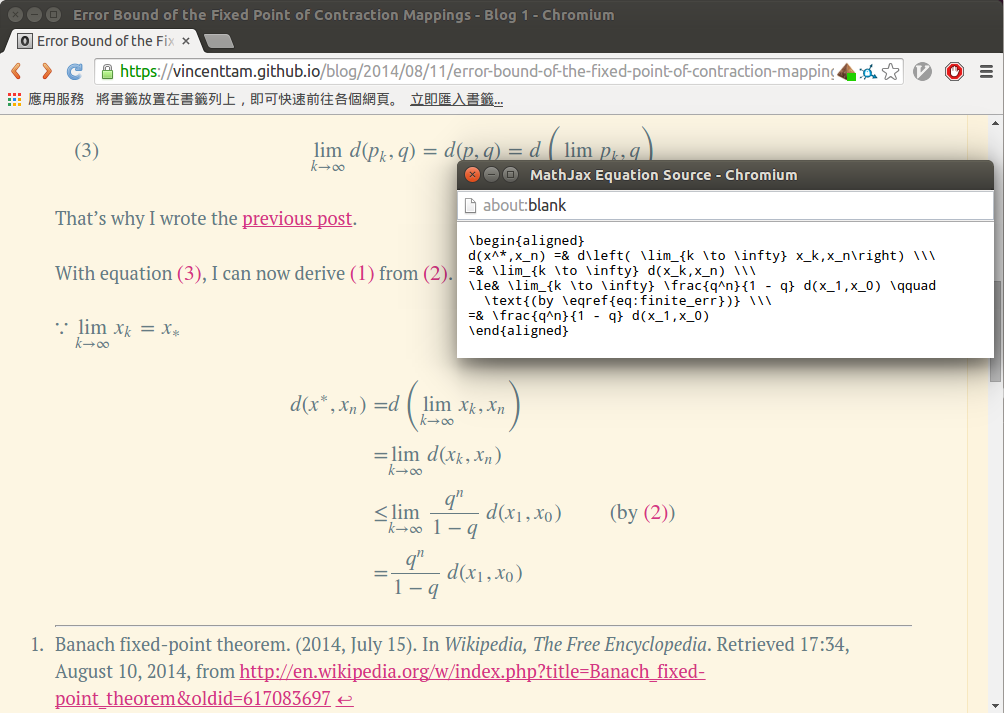Visual effects in pages under “/posts/” or the index page

Note that due to the development of Octopress, I now see three backslashes in the “MathJax Equation Source”.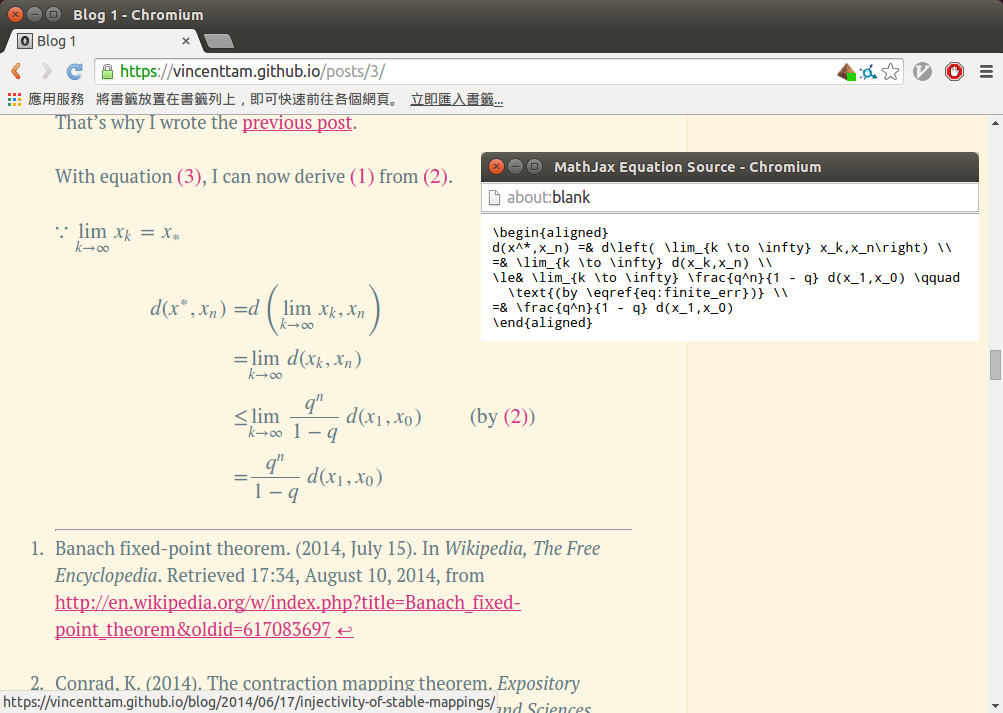It doesn’t matter much in block equations.

Problem

However, it does matter if I have to define a piecewise function. Take one defined in one of my old posts as an example.3

(Added on DEC 12TH, 2014) (Revised on SEP 3RD, 2015)

Note: As you can see from the above piecewise function, the problem is now gone.

$f(x,y) = \begin{cases} 0 &\text{if } (x,y) \in \vect{I} \land y \ge x\\\ 1 &\text{if } (x,y) \in \vect{I} \land y < x \end{cases}$

Visual effects in a page for a single postVisual effects in pages under “/posts/” or the index page

Note that due to the development of Octopress, I now see three backslashes in the “MathJax Equation Source”.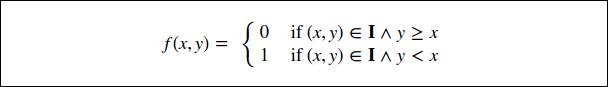Solution

Now, I know how to tell kramdown to ignore MathJax code. This is much more convenient than the method described below.

After I observed that the two equations, which are aligned by the aligned environment, at the bottom of the post cited in footnote #3, I used the $\rm \LaTeX$ commands \left\\{ and \right. in the Markdown source file for posts to construct the left curly brace only.

f(x,y) = \left\{ \begin{aligned} 0 &\text{ if } (x,y) \in \vect{I} \land y \ge x\\\ 1 &\text{ if } (x,y) \in \vect{I} \land y < x, \end{aligned} \right.

Visual effects in a page for a single post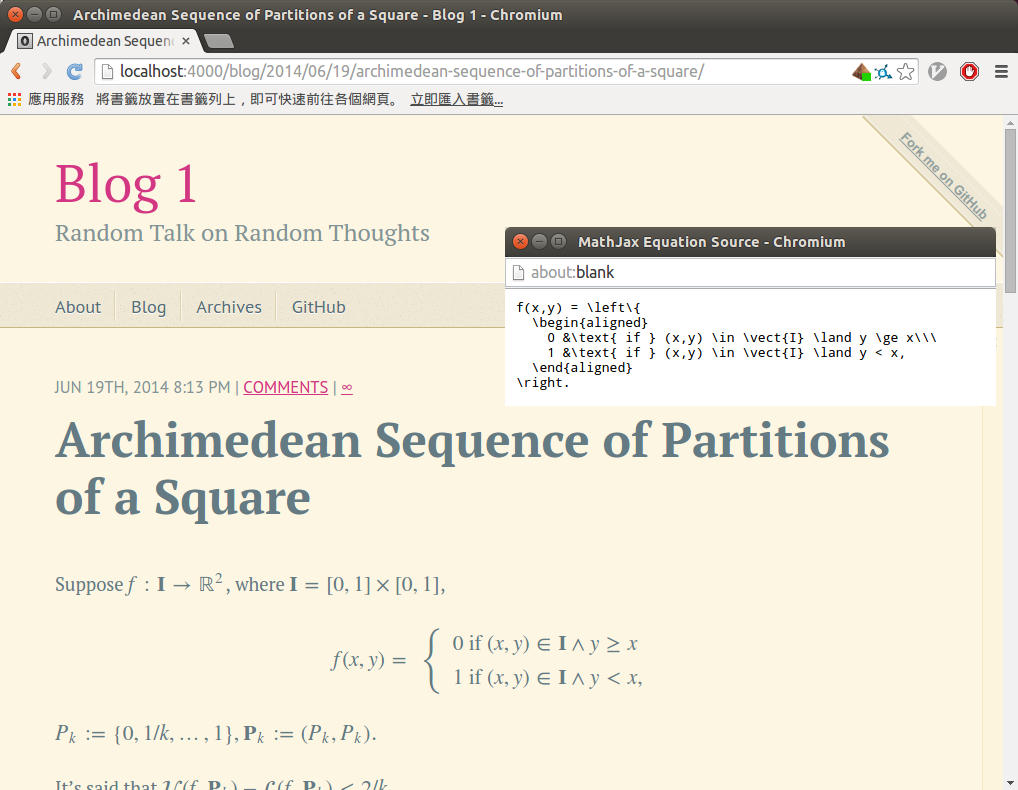Visual effects in pages under “/posts/” or the index page

Note that due to the development of Octopress, I now see three backslashes in the “MathJax Equation Source”.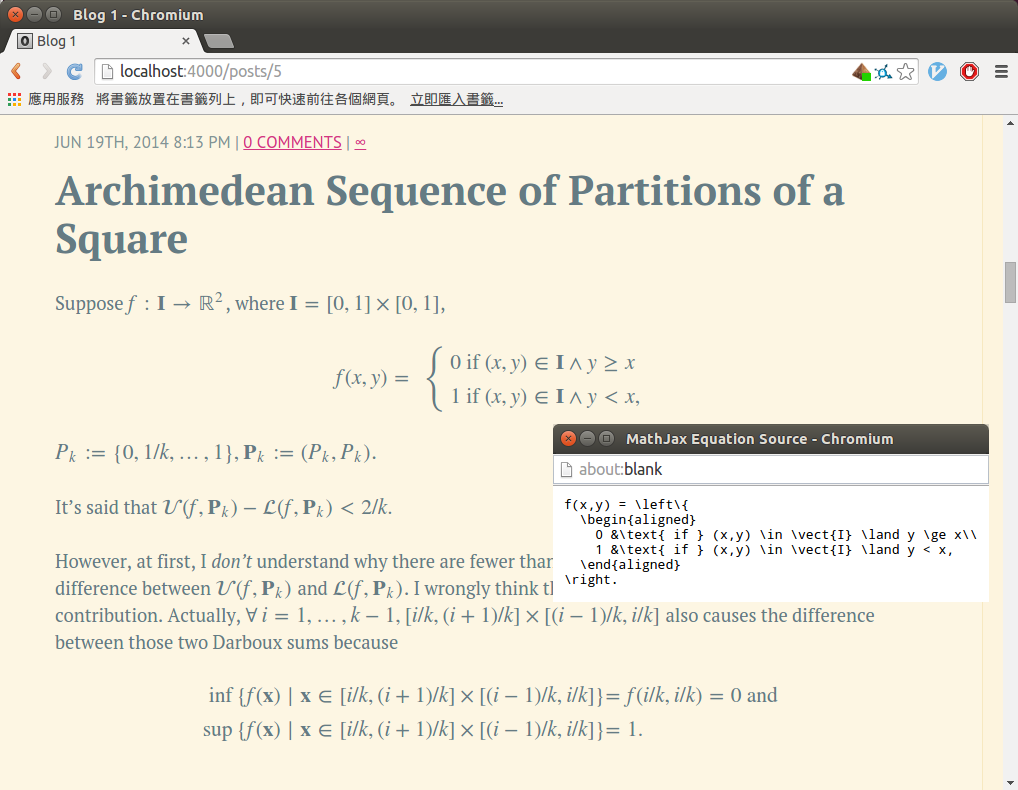‘0’ and ‘1’ in the above example are aligned. Though in pages for each individual posts, the whitespace character preceeding ‘1’ still exists, it isn’t visible unless it’s highlighted. I’m quite satified with this alternative method of typesetting piecewise functions.

1. See MathJax and RSS in Blog 1 for details.

2. See Error Bound of the Fixed Point of Contraction Mappings in Blog 1 for details.

3. See Archimedean Sequence of Partitions of a Square in Blog 1 for details.